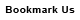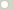# kilogram-force meter (Torque, Metric)

## 1 kilogram-force meter is equal to:Metric newton meter 9.80665012480924 newton centimeter 980.665012480924 dyne meter 980665.012480924 dyne centimeter 98066501.2480924 kilogram-force centimeter 100 gram-force meter 1000 gram-force centimeter 100000 British and U.S. long ton-force foot 0.003229024 short ton-force foot 0.0036165069 pound-force foot 7.2330139 pound-force inch 86.796166 ounce-force inch 1388.7387ConversionsAreaCapacity and VolumeCircular measureComputer storageCurrencyDistance and LengthEnergy and WorkFuel ConsumptionMass and WeightPowerPressureSpeedTemperatureTimeTorqueSearchLink to us